Scalar product - Defination

Chapter 10 Class 12 Vector Algebra
Concept wise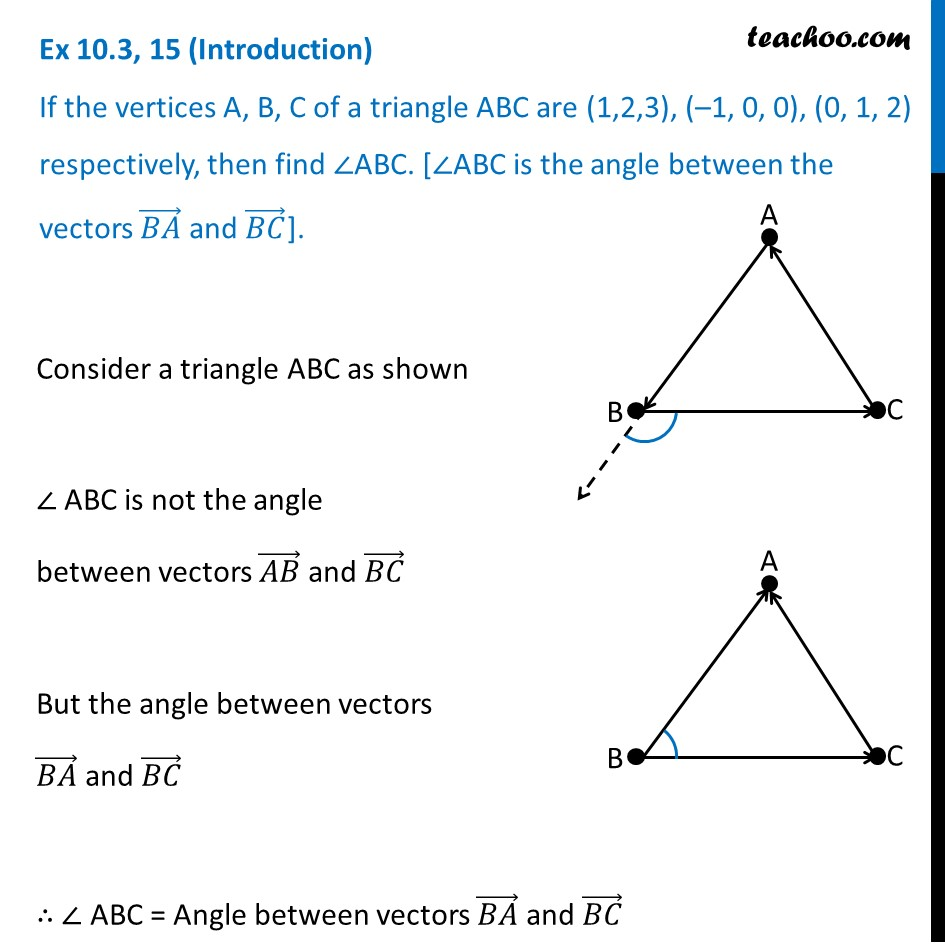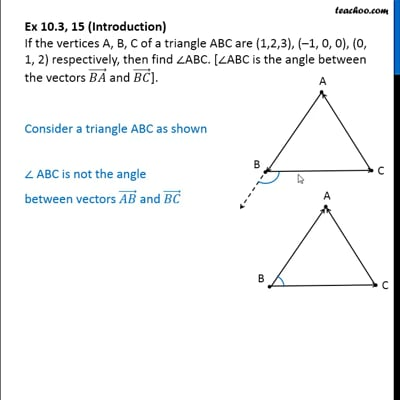This video is only available for Teachoo black users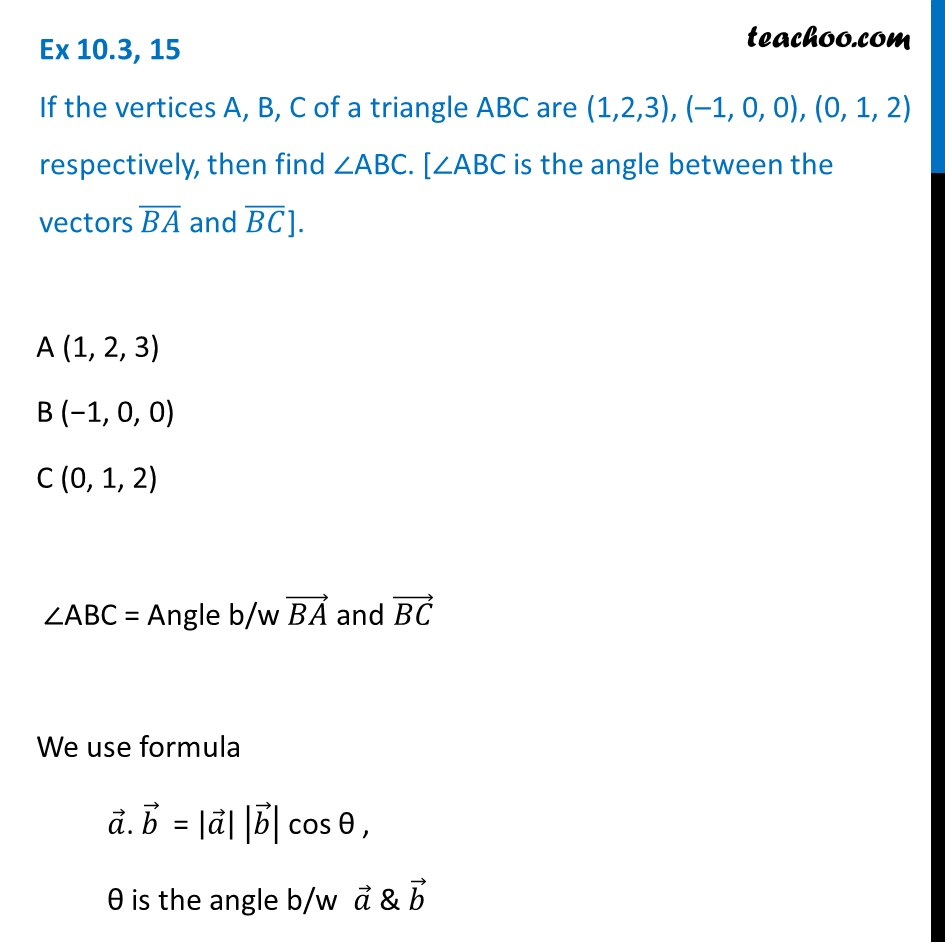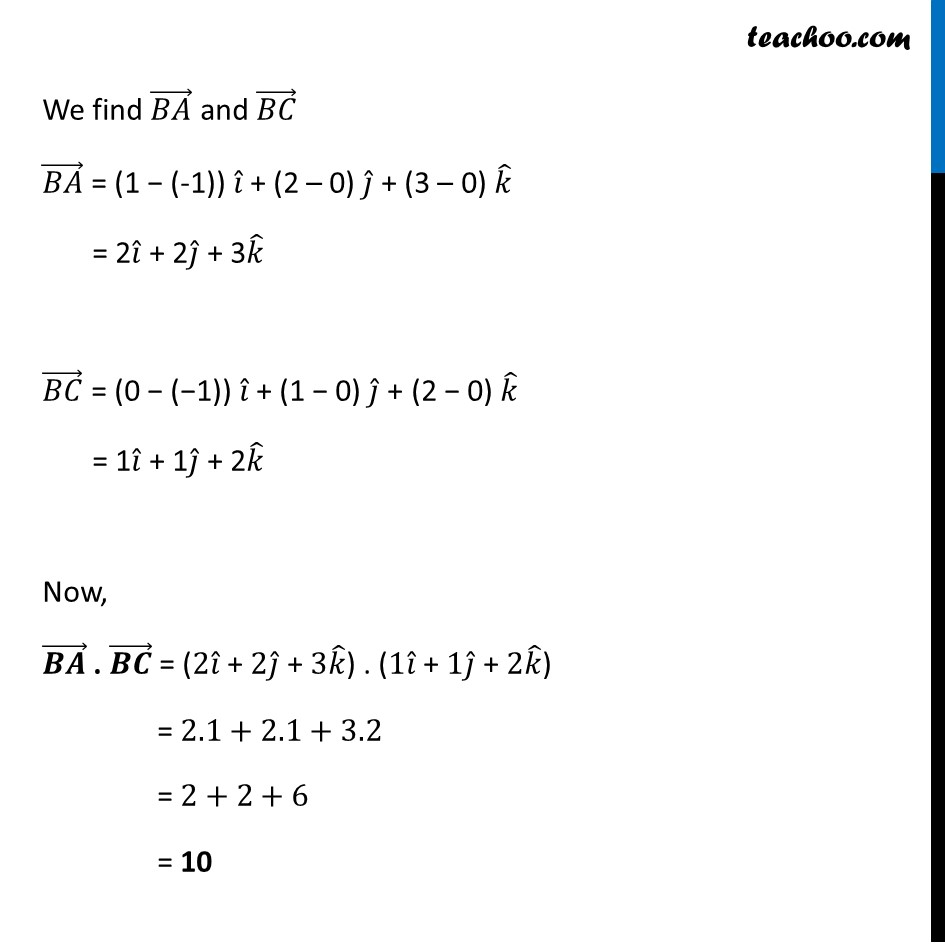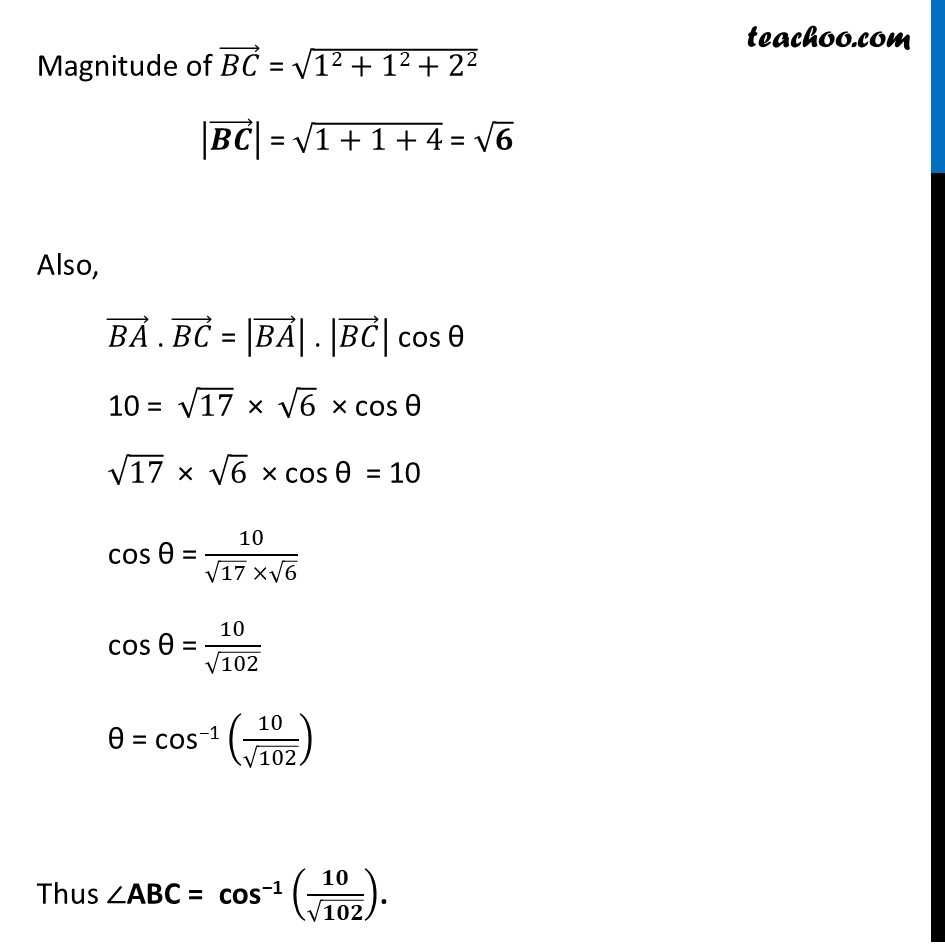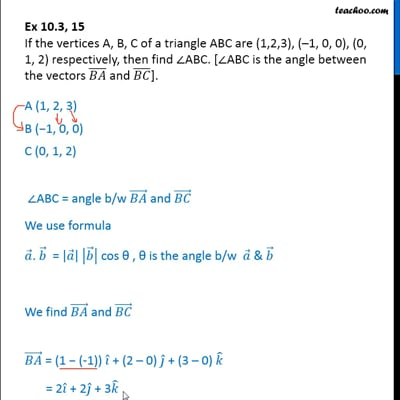This video is only available for Teachoo black users

Introducing your new favourite teacher - Teachoo Black, at only ₹83 per month

### Transcript

Ex 10.3, 15 (Introduction) If the vertices A, B, C of a triangle ABC are (1,2,3), (–1, 0, 0), (0, 1, 2) respectively, then find ∠ABC. [∠ABC is the angle between the vectors (𝐵𝐴) ⃗ and (𝐵𝐶) ⃗]. Consider a triangle ABC as shown ∠ ABC is not the angle between vectors (𝐴𝐵) ⃗ and (𝐵𝐶) ⃗ But the angle between vectors (𝐵𝐴) ⃗ and (𝐵𝐶) ⃗ ∴ ∠ ABC = Angle between vectors (𝐵𝐴) ⃗ and (𝐵𝐶) ⃗ Ex 10.3, 15 If the vertices A, B, C of a triangle ABC are (1,2,3), (–1, 0, 0), (0, 1, 2) respectively, then find ∠ABC. [∠ABC is the angle between the vectors (𝐵𝐴) ̅ and (𝐵𝐶) ̅]. A (1, 2, 3) B (−1, 0, 0) C (0, 1, 2) ∠ABC = Angle b/w (𝐵𝐴) ⃗ and (𝐵𝐶) ⃗ We use formula 𝑎 ⃗. 𝑏 ⃗ = |𝑎 ⃗ | |𝑏 ⃗ | cos θ , θ is the angle b/w 𝑎 ⃗ & 𝑏 ⃗ We find (𝐵𝐴) ⃗ and (𝐵𝐶) ⃗ (𝐵𝐴) ⃗ = (1 − (-1)) 𝑖 ̂ + (2 – 0) 𝑗 ̂ + (3 – 0) 𝑘 ̂ = 2𝑖 ̂ + 2𝑗 ̂ + 3𝑘 ̂ (𝐵𝐶) ⃗ = (0 − (−1)) 𝑖 ̂ + (1 − 0) 𝑗 ̂ + (2 − 0) 𝑘 ̂ = 1𝑖 ̂ + 1𝑗 ̂ + 2𝑘 ̂ Now, (𝑩𝑨) ⃗ . (𝑩𝑪) ⃗ = ("2" 𝑖 ̂" + " 2𝑗 ̂" + " 3𝑘 ̂) . ("1" 𝑖 ̂" + " 1𝑗 ̂" + " 2𝑘 ̂) = 2.1+2.1+3.2 = 2+2+6 = 10 Magnitude of (𝐵𝐶) ⃗ = √(12+12+22) |(𝑩𝑪) ⃗ | = √(1+1+4) = √𝟔 Also, (𝐵𝐴) ⃗ . (𝐵𝐶) ⃗ = |(𝐵𝐴) ⃗ | . |(𝐵𝐶) ⃗ | cos θ 10 = √17 × √6 × cos θ √17 × √6 × cos θ = 10 cos θ = 10/(√17 ×√6) cos θ = 10/√102 θ = cos−1 (10/√102) Thus ∠ABC = cos−1 (𝟏𝟎/√𝟏𝟎𝟐).